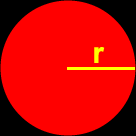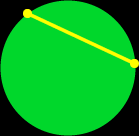# The Geometry of Circles

Definitions and formulas for the radius of a circle, the diameter of a circle, the circumference (perimeter) of a circle, the area of a circle, the chord of a circle, arc and the arc length of a circle, sector and the area of the sector of a circle
Just scroll down or click on what you want and I'll scroll down for you!

 radius of a circle diameter of a circle circumference of a circle area of a circle chord of a circle arc of a circle arc length of a circle sector of a circle area of a sector of a circle

 The radius of a circle:The radius of a circle is the distance from the center of the circle to the outside edge.The diameter of a circle:The diameter of a circle is longest distance across a circle.  (The diameter cuts through the center of the circle.  This is what makes it the longest distance.)The circumference of a circle (the perimeter of a circle):The circumference of a circle is the perimeter -- the distance around the outer edge.Circumference =where r = the radius of the circleand pi = 3.141592...The area of a circle:Area =where r = the radius of the circleand pi = 3.141592...A chord of a circle:A chord of a circle is a line segment that connects one point on the edge of the circle with another point on the circle.(The diameter is a chord -- it's just the longest chord!)